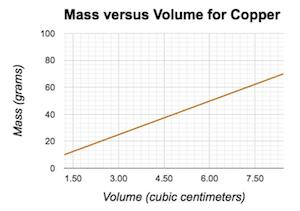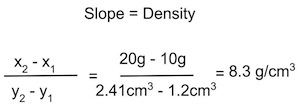# Calculating Density with Mass vs. Volume Graphs

An error occurred trying to load this video.

Try refreshing the page, or contact customer support.

Coming up next: The Atom

### You're on a roll. Keep up the good work!

Replay
Your next lesson will play in 10 seconds
• 0:00 What Is Density?
• 0:56 Mass vs. Volume Graphs
• 1:45 Comparing Density
• 2:09 Identifying Substances
• 3:51 Lesson Summary

Want to watch this again later?

Timeline
Autoplay
Autoplay
Speed

#### Recommended Lessons and Courses for You

Lesson Transcript
Instructor: Amanda Robb
In this lesson, we'll learn what density is and how to calculate it. We'll also learn how to graph mass versus volume to find density for different objects.

## What Is Density?

Picture dropping a penny and a bottle cork into a pond. You'd probably expect the penny to sink, even though it's smaller in size than the cork, but why? It has to do with density, or the amount of mass contained in a certain volume. If something has a greater density, like the penny, it means there is more matter in the same amount of volume. The penny is more dense than the cork and water, so it sinks. Although larger than the penny, the cork is less dense than the penny. It's also less dense than the water, so it floats. But without this experiment, how can we figure out which object is denser?

Density is equal to mass divided by volume (D = m / v), so if you know the mass and volume of an object, you can calculate density. Sometimes, you'll be given this information outright, but other times you might be given a graph of mass versus volume. Let's learn how you can use the information in this type of graph to calculate density.

## Mass vs. Volume Graphs

A mass versus volume graph has mass, usually in grams or kilograms, on the y-axis, which is the vertical axis, and volume on the x-axis, which is the horizontal access.

You can use this graph to figure out how much mass is in any given volume of a substance. Using the x-axis, locate the volume you're wondering about, then find the y-intercept and use the y-axis to find how much mass there is in that much volume. For example, if we had a sample of copper with a volume of six cubic centimeters, based on the mass versus volume graph, the mass would be 50 grams.The formula for the slope of a straight line is the change in y divided by the change in x. Since the y axis is equal to mass, and the x axis is equal to volume, slope is equal to mass divided by volume. Therefore, the slope of a mass versus volume graph is equal to density.## Comparing Density

Since slope is equal to density, simply glancing at a mass versus volume graph can sometimes help you identify which of the two substances has a greater density. A steeper line indicates a greater slope and thus a greater density. So, whichever substance has a steeper line has a greater density. You can also use the calculations we just discussed to find the density of a substance. We'll do this in an example in the next section of the lesson.

To unlock this lesson you must be a Study.com Member.

### Register to view this lesson

Are you a student or a teacher?

### Unlock Your Education

#### See for yourself why 30 million people use Study.com

##### Become a Study.com member and start learning now.
Back
What teachers are saying about Study.com

### Earning College Credit

Did you know… We have over 160 college courses that prepare you to earn credit by exam that is accepted by over 1,500 colleges and universities. You can test out of the first two years of college and save thousands off your degree. Anyone can earn credit-by-exam regardless of age or education level.